# The metric dimension of strong product graphs

### Abstractcarpathian_2015_31_2_261_268_abstract

### Full PDFcarpathian_2015_31_2_261_268

Issue no:

For an ordered subset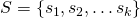of vertices in a connected graph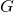, the metric representation of a vertex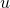with respect to the set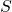is the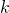-vector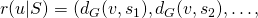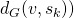, where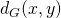represents the distance between the vertices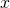and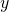. The setis a metric generator forif every two different vertices ofhave distinct metric representations with respect to. A minimum metric generator is called a metric basis forand its cardinality,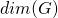, the metric dimension of. It is well known that the problem of finding the metric dimension of a graph is NP-Hard. In this paper we obtain closed formulae and tight bounds for the metric dimension of strong product graphs.